# Incircle geometry

The incircle of triangle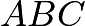touches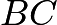,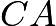and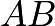at pointsandrespectively. Let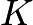be a point such that.Prove that the perpendicular from incenterto the,and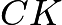are concurrent.

2 Likes

@Gomuj hey bhai, a geo problem. Can you help him?

1 Like

@Tahmid_S can you help?

Nice problem! Here’s my solution:

Let X be the intersections of line A'B' and the perpendicular from I on CM. Since A'B' is the polar of C, CM must be the polar of X. Let Y=\overline{A'B'}\cap\overline{CM}. Then (A', B'; X, Y) = -1. Suppose P_\infty is the point at infinity along line AB. Then we have -1 = (B, A; P_\infty, M) \overset{C}{=} (A', B'; \overline{CK}\cap\overline{A'B'}, Y). Therefore X = \overline{CK}\cap\overline{A'B'}, and we are done. \blacksquare

Btw, it seems I can’t write maths in display mode. Why is that?

3 Likes